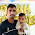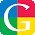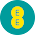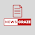### E.M.F. Equation of D.C. Generator

Let       P = Number of poles of the generator
Φ = Flux produced by each pole in webers (Wb)
N = Speed of armature in r.p.m.
Z = Total Number of Armature Conductors
A = Number of parallel paths in which the 'Z' number of conductors are divided
So        A = P for lap type of winding
A = 2 for wave type of winding
Now e.m.f. gets induced in the conductor according to Faraday's law of electromagnetic induction. Hence average value of e.m.f. induced in each armature conductor is,
e = Rate of cutting the flux = dΦ/dt
Now consider one revolution of conductor. In one revolution, conductor will cut total flux produced by all the poles i.e. Φ x P. While time required to complete one revolution is 60/N seconds as speed is N r.p.m.

This is the e.m.f. induced in one conductor. Now the conductors in one parallel path are always in series. There are total Z conductor with A parallel paths, hence Z/A number of conductors are always in series and e.m.f. remains same across all the parallel paths.
...     Total e.m.f. can be expressed as,

This is nothing but the e.m.f. equation of a d.c. generator.

Example : A 4 pole, lap wound, d.c. generator has a useful flux of 0.07 Wb per pole. Calculate the generated e.m.f. when it is rotated at a speed of 900 r.p.m. with the help of prime mover. Armature consists of 440 number of conductors. Also calculate the generated e.m.f. if lap wound armature is replaced by wave wound armature.
Solution :
P = 4    Z = 440   Φ = 0.07 Wb    and   N = 900 r.p.m.

i) For lap wound,      A = P = 4

ii) For wave wound    A = 2

1.Great Explanation

2.Are you looking for electrical online study site. Visit Electrical Interview Questions And tutorial

3.i found more simple way here dc generator emf equation

4.5.6.